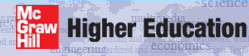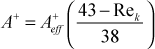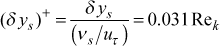# Institut für Thermodynamik der Luft- und Raumfahrt - Universität StuttgartMechanical Engineering - The University of Texas at Austin# roughness

The roughness effects in academic TEXSTAN are limited to the mixing length model and the one-equation model, which also uses the mixing length. The model is developed in detail in our textbook CHMT. The model is valid only for the class of roughness called the Nikuradse uniformly distributed roughness, which can be characterized by two variables, the roughness length scale ks and the roughness Reynolds number, Rek = ks /(ν/uτ). For this model the roughness length scale is introduced using the input variable cxx for the E-surface (kgeom=4,5,6,7). Note, for kgeom=6,7, where there are two walls, and the input variable gxx is used to specify the roughness length scale for the second surface, the I-surface. The roughness model is switched on for the mixing length in academic TEXSTAN by setting k9=4.

Note, to monitor the computation of the roughness model, the roughness variables are written to a TEXSTAN output file ftn93.txt.

For Rek ≤ 5, the surface is considered smooth and no mixing-length modifications are applied. For 5 < Rek ≤ 43 , the roughness effect is simulated by reducing the A+ sublayer constant in the van Driest model using the linear functionand for Rek > 43 the sublayer vanishes, A+=0, and a mixing length offset is applied using the ideas described in CHMT,where δys is proportional to ks but considerably smaller. The model for it is taken from experimental data, where the wall-coordinate offset correlates with the roughness Reynolds numberFor flow over extremely rough surfaces, the auto-generated initial profiles for velocity and temperature can be constructed as flat profiles using kstart=9 and by setting the flag k9 >0 and providing the estimated velocity profile height as the input variable gxx.

website updated Sept 2010   © 1996-2010 Michael E. Crawford - all rights reserved - website validated for CSS 2.1 and XHTML 1.0 strict at www.w3.org